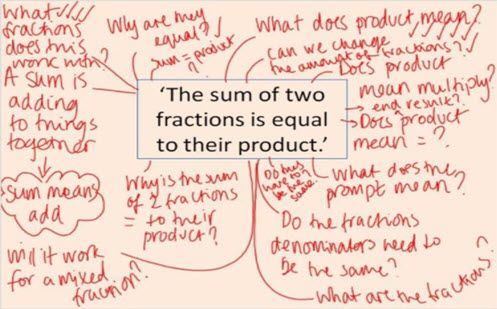Go to website

# Sum and product

This prompt sets up a problem and the students have to find two fractions that will fit the requirements. Students need to understand the vocabulary in the question. The resource includes the prompt, teacher notes, guided questions and extension prompts.

Year level(s) Year 7
Audience Teacher
Teaching strategies and pedagogical approaches Collaborative learning, Differentiated teaching, Explicit teaching, Feedback, Mathematics investigation
Keywords fraction, product, sum

## Curriculum alignment

Curriculum connections Critical and creative thinking, Numeracy
Strand and focus Number, Build understanding, Apply understanding
Topics Multiplication and division, Fractions
AC: Mathematics (V9.0) content descriptions
AC9M7N06
Use the 4 operations with positive rational numbers including fractions, decimals and percentages to solve problems using efficient calculation strategies

Interpreting fractions (P8)
Multiplicative strategies (P10)
Number and place value (P10)
Proportional thinking (P2)